Ex 6.3

Chapter 6 Class 6 Integers
Serial order wise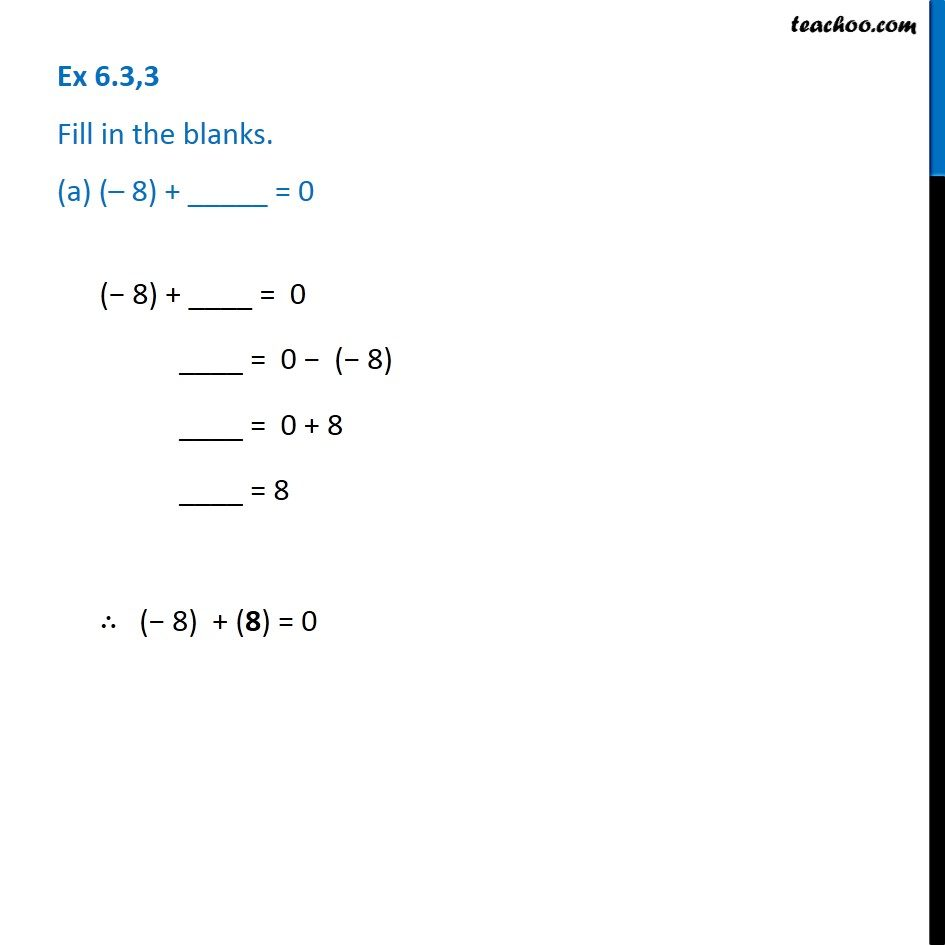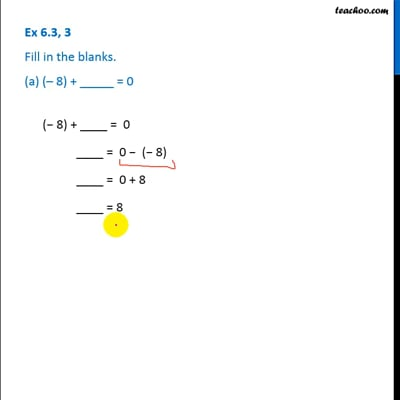This video is only available for Teachoo black users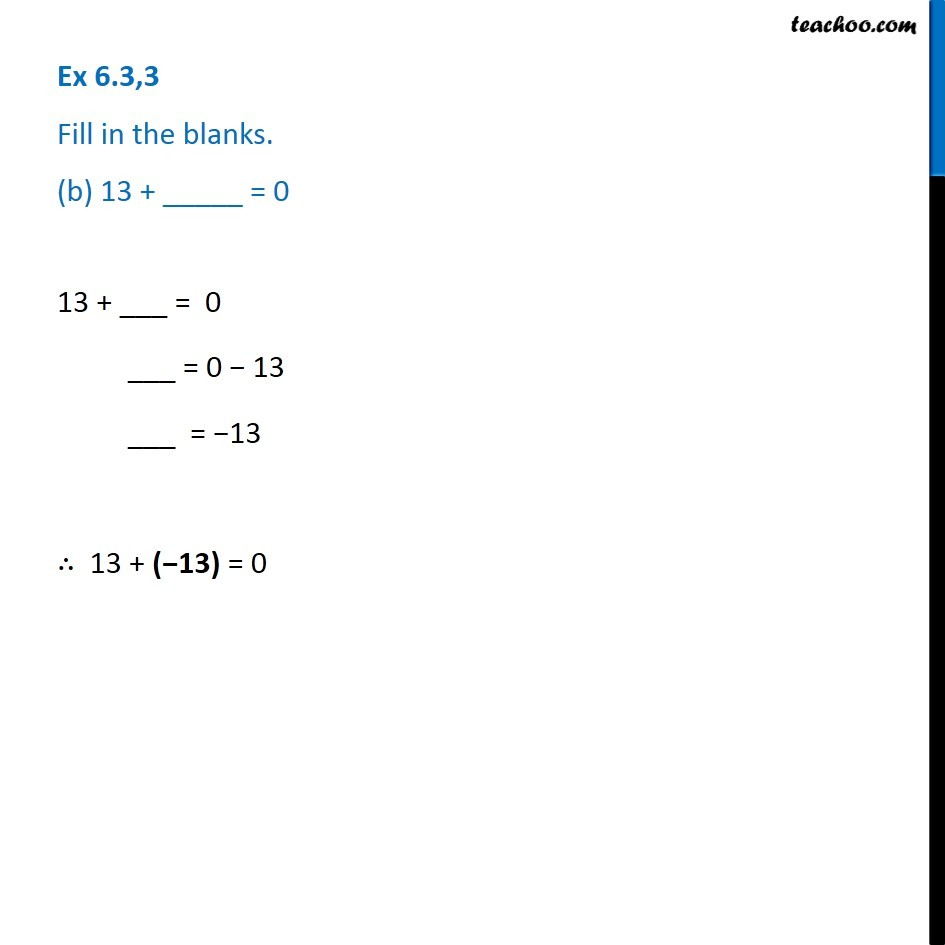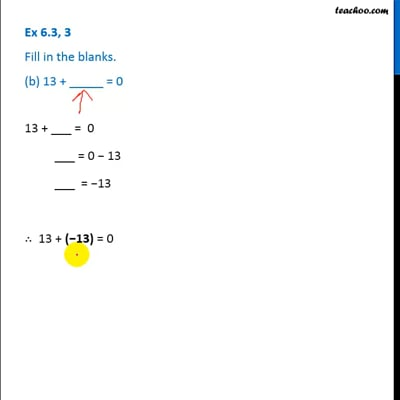This video is only available for Teachoo black users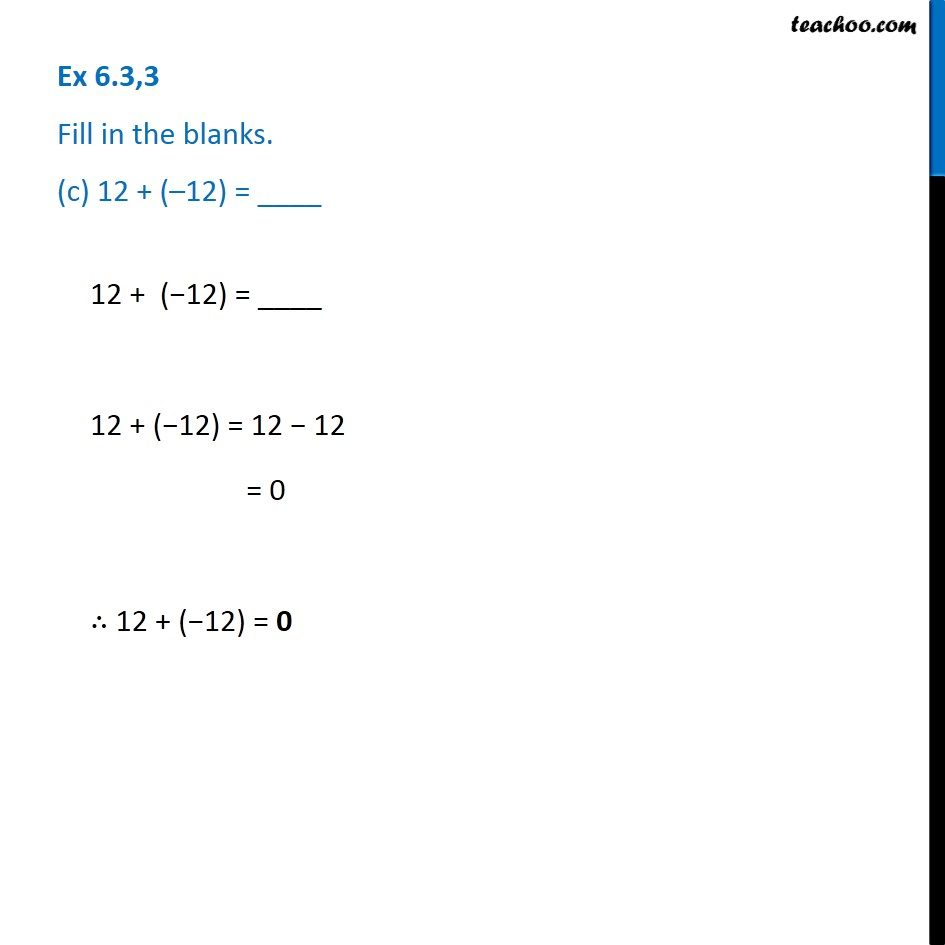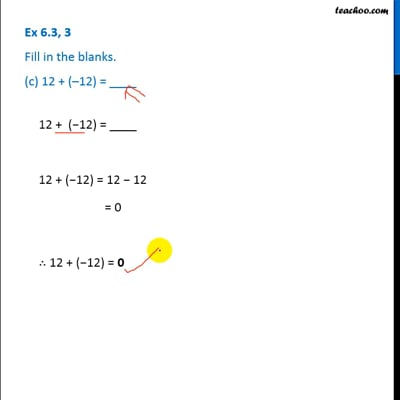This video is only available for Teachoo black users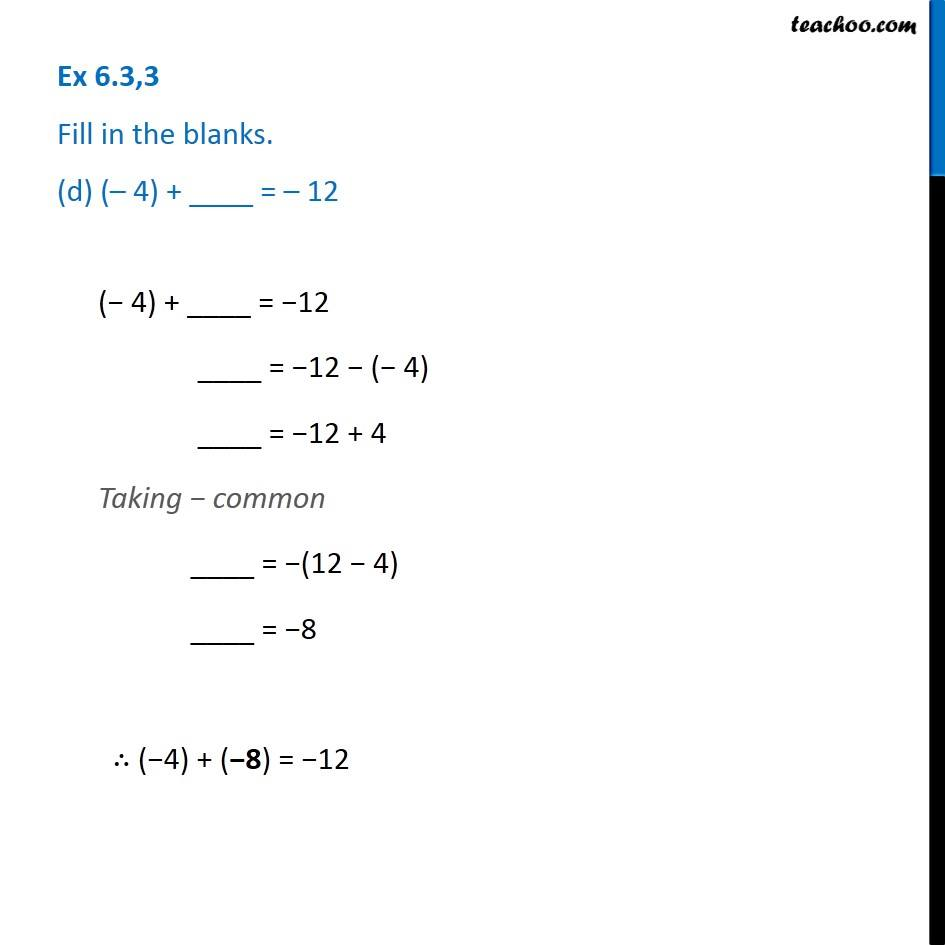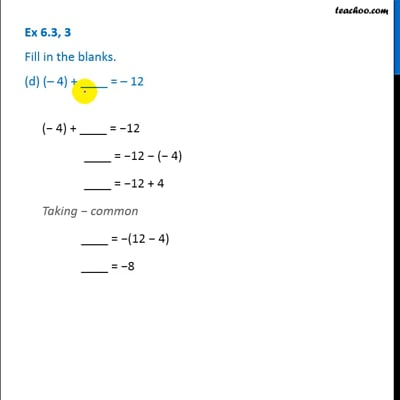This video is only available for Teachoo black users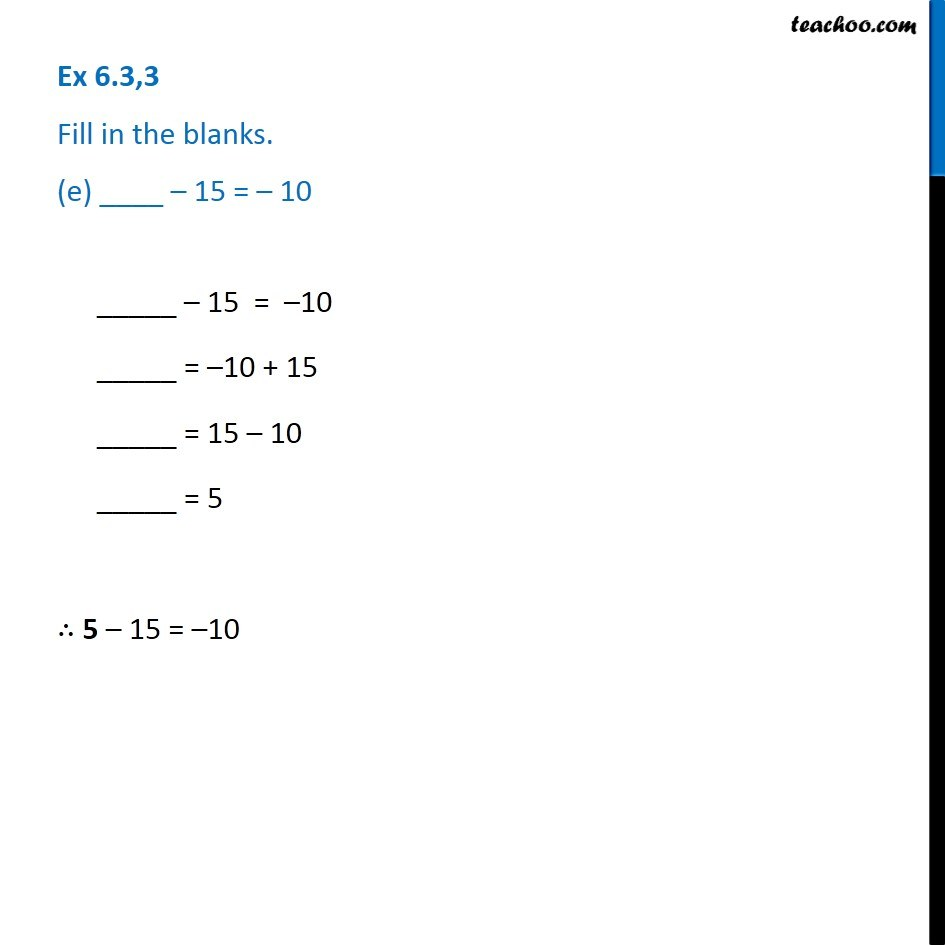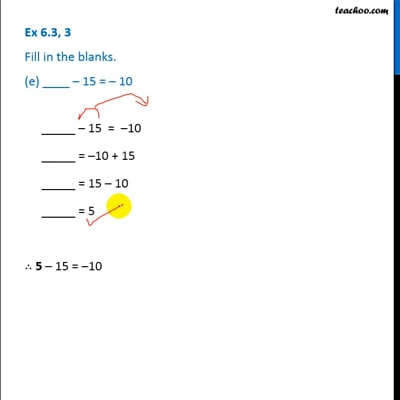This video is only available for Teachoo black users

### Transcript

Ex 6.3,3 Fill in the blanks. (a) (– 8) + _____ = 0(− 8) + ____ = 0 ____ = 0 − (− 8) ____ = 0 + 8 ____ = 8 ∴ (− 8) + (8) = 0 Ex 6.3,3 Fill in the blanks. (b) 13 + _____ = 0 13 + ___ = 0 ___ = 0 − 13 ___ = −13 ∴ 13 + (−13) = 0 Ex 6.3,3 Fill in the blanks. (c) 12 + (–12) = ____ 12 + (−12) = ____ 12 + (−12) = 12 − 12 = 0 ∴ 12 + (−12) = 0 Ex 6.3,3 Fill in the blanks. (d) (– 4) + ____ = – 12 (− 4) + ____ = −12 ____ = −12 − (− 4) ____ = −12 + 4 Taking − common ____ = −(12 − 4) ____ = −8 ∴ (−4) + (−8) = −12 Ex 6.3,3 Fill in the blanks. (e) ____ – 15 = – 10 _____ – 15 = –10 _____ = –10 + 15 _____ = 15 – 10 _____ = 5 ∴ 5 – 15 = –10